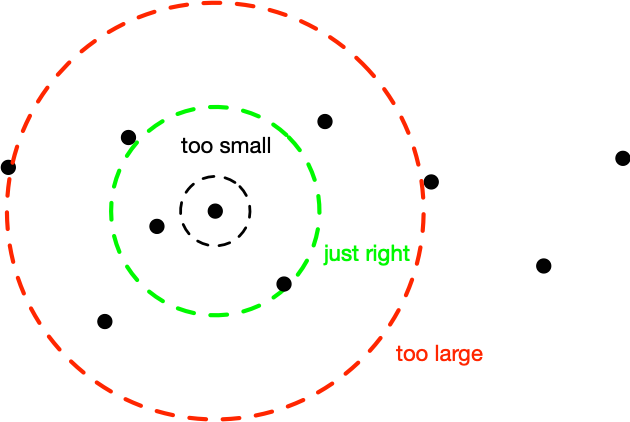# Easy Kernel Width Selection

kernel
trick
model selection
Published

October 12, 2010This is an idea that was originally put forward by Bernhard Schölkopf in his thesis: Assume you have an RBF (radial basis function) kernel and you want to know how to scale it. Recall that such a kernel is given by

$k(x,x') = \kappa(\lambda \|x - x'\|)$

For instance, Gaussian RBFs can be written as $$k(x,x') = \exp(-\lambda^2 \|x-x'\|^2)$$. We want that the argument of this function is $$O(1)$$ for typical pairs of instances $$x$$ and $$x’$$. Bernhard proposed to look at the dimensionality of x and rescale accordingly. This is a great heuristic. But it ignores correlation between the coordinates. A much simpler trick is to pick, say 1000 pairs $$(x,x’)$$ at random from your dataset, compute the distance of all such pairs and take the median, the $$0.1$$ and the $$0.9$$ quantile. Now pick $$\lambda$$ to be the inverse any of these three numbers. With a little bit of crossvalidation you will figure out which one of the three is best. In most cases you won’t need to search any further.